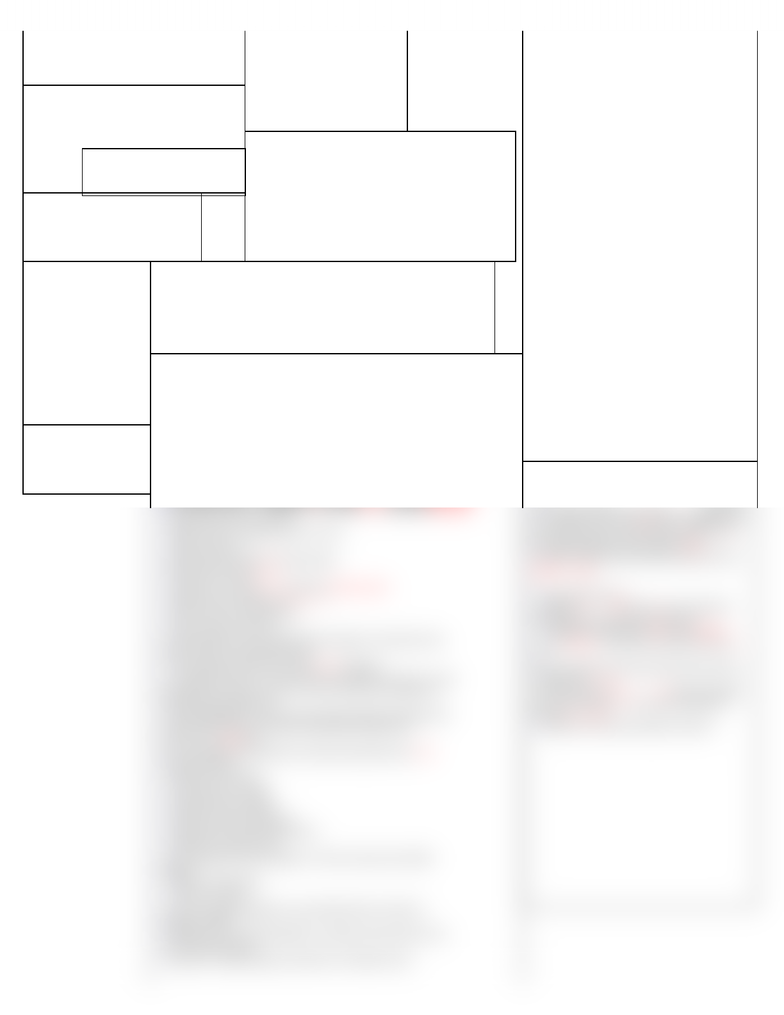Class Notes (1,200,000)
CA (650,000)
UW (20,000)
CS (1,000)
CS240 (60)
Lecture

# CS240 Lecture Notes - Avl Tree, Feta, Quicksort

Department
Computer Science
Course Code
CS240
Professor
Therese Biedl

This preview shows half of the first page. to view the full 1 pages of the document.Bubble-up (Node i ):Time = O(logn) use 4 insert
while (p(i ) exists) and (value(p(i)) < value(i)) {
exchange values(i ) and value(p(i ));
i ← p(i );}
Bubble-down (Node i ): Time:O(logn) use EMax
while (i has children) {
let j be the child of i with the largest value
if value(j) > value(i ) then
exchange values(i ) and value(j);
i ← j ;
otherwise
break;}
Heapsort /* Heapify: */Equal(n)
for i := n/2 downto 1
Bubble-down(i)
/* ExtractMax one at a time */
while (n > 1) {
Swap(A[n], A);
n--;
Bubble-down(1);}
QuickSort(int[] A, int left, int right):
if left < right
int i := Partition(A, left, right);
QuickSort(A, left, i-1);
QuickSort(A, i+1, right);
Partition(A, left, right):
pivot := A[right];
i := left-1;
for j := left to right
if A[j] <= pivot
i := i+1
swap(A[j], A[i])
return i;
Running time of Partition:
Equal(right − left + 2).
Auxiliary Space: Equal(1).
QuickSortRecurseOnSmallerPart(int[] A, int left, int right):
int myLeft := left, myRight := right;
while (myLeft < myRight) do
| int i := Partition(A, myLeft, myRight);
| if (i-myLeft) < (myRight - i) then
| | QuickSortRecurseOnSmallerPart(A, myLeft, i-1);
| | myLeft = i+1
| else
| | QuickSortRecurseOnSmallerPart(A, i+1, myRight);
| | myRight = i-1
RandomPermute(A, n):
for i ← 1 to n
exchange(A[i ], A[random(i , n)])
Theorem
The height of an AVL tree with n nodes is O(log n).
Proof: What is the least number of nodes (nh) of an AVL-tree of height h?
Recurrence relation: ni = ni−1 + ni−2 + 1.
ni = Fi+3 1 = floor( feta^(i+3) / sqrt(5) ) + ½ - 1
Therefore, ni = equal( feta^I )
Hence, n ≥ nh = equal(feta^I.) implies h = O(logn)
Extendable hashing: search
Given a key k, compute h(k) = a1a2 . . . aL.
Probe block B(a1 . . . ad ).
Perform a binary search to find all items in the block.
If the directly resides in internal memory, we have only a single memory
block transfer.
Insert(x) is done as follows:
Search for x to find the proper block to insert
If the block has space then done
If the block is full (i.e. has already S keys) and k < d, perform ablock split:
Split the block into two blocks.
Separate items according to the (k+1)st bit
Update references
Increase the local depth k in those blocks.
Repeat until room or k = d.
If the block is full and k = d, perform a directory split
double the size of the direct (d = d + 1)
Update references appropriately.
back to the previous cases.
The hash table is much smaller than the number of stored keys and
therefore may fit in the main memory.
Most operations should access only O (1) leaf pages.
To make more space, we only add a block. Rarely do we have to copy
the hash table. Never do we have to move all items in dictionary (in
main disadvantage: we use more space than actually needed (but one
can show that if keys are uniformly distributed then pages are
expected to be %69 full).
We can implement dictionaries such that all operations take O(1)
expected run time.
Hashing with chaining:
no explicit need to rehash
keep load factor much smaller than 1.
rehashing is required often.
fast computation (linear hashing) vs. better average time (double
hashing)
deletion is problematic.
no space is wasted.
We are strongly depending on a good hash function, otherwise
hashing is terrible.
Hashing can be extended naturally to situations where data does not
reside in main memory.
In practice, usually hashing is preferred over balanced trees.
Priority queue sort
for i 1 . . . n do
PQ.insert(A[i]);
for i n . . . 1 do
A[i] = PQ.extractMax();